### Italian coins Repubblica

Description and catalogue value of italian coins 1943 - 2001.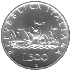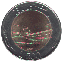### Italian coins Vittorio Emanuele III

here you can find a catalogue of
Vittorio Emanuele III coins.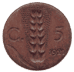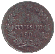### Error Euro

If you are interested in some Euro errors of the European Mints.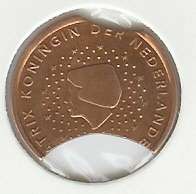All the information about errors I've found on the net about the Euro.

### Italian Euro

A Short description about the Italian Euro.

### Vatican Euro

A Short description about the Vatican Euro.

### Telephone counter - Gettone telefonico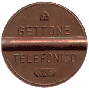.

### Tools for Numismatic and Currency

World currency converter
Euro Currency converter
Scanner Images

.
.
.
.
.
.
.
.
.

# How to Calculate the Specific Gravity of a Coin

[ITA]         [ENG]

## Manual

### Why?

To Know the specific gravity of a coin it is useful for the determination of its gold or silver content, this is an useful information to discover some fakes or to study the composition of certain coins.
Knowing the weight and volume it is possible to determine the specific gravity (SG) of the coin, moreover if the alloy components are known it is possible to determine with good precision the relative weight. Often the purity of the metal is a good sign of authenticity.
If we want to use a simple measure of weight in order to identify a false coin is not a procedure that can always be right, because the weight is the physical parameter easier to measure, and therefore it is reproduced with absolute accuracy by forgers.

### How

The experiment exploits the physical principle that a body immersed in a liquid increases the extent of the liquid volume by an amount equal to the volume of the body. If we know the specific weight of the liquid we can determine the volume of the body and therefore its specific gravity.
We take our "guinea pig" for this experiment: a Vittorio Emanuele II scudo 1874.
The steps are as follows:
• 1 - calibrate the scale (in this case was used a 100g step 0.01 g)
• 2 - control of the calibration (perfectly ok)
• 3 - first it is need to weigh the coin "dry", the coin shows no obvious signs of dust, only a slight patina.
measured weight 24.89 grams.
• 4 - construction of the suspension system for the coin
in the following pictures you can see the suspension system built with pieces of a wooden fruit box, the wire is an ordinary sewing thread, it is important that this should be as light as possible.
I tried to weigh the wire in water but, after carefully dipped a piece of wire 20 times greater than that we're going to immerse with the coin, I hadn't a considerable measure, so the wire immersed give as difference in weight less than 5% of 0,01 gram. We can consider its weight negligible.

In the images below it is possible to see the suspension system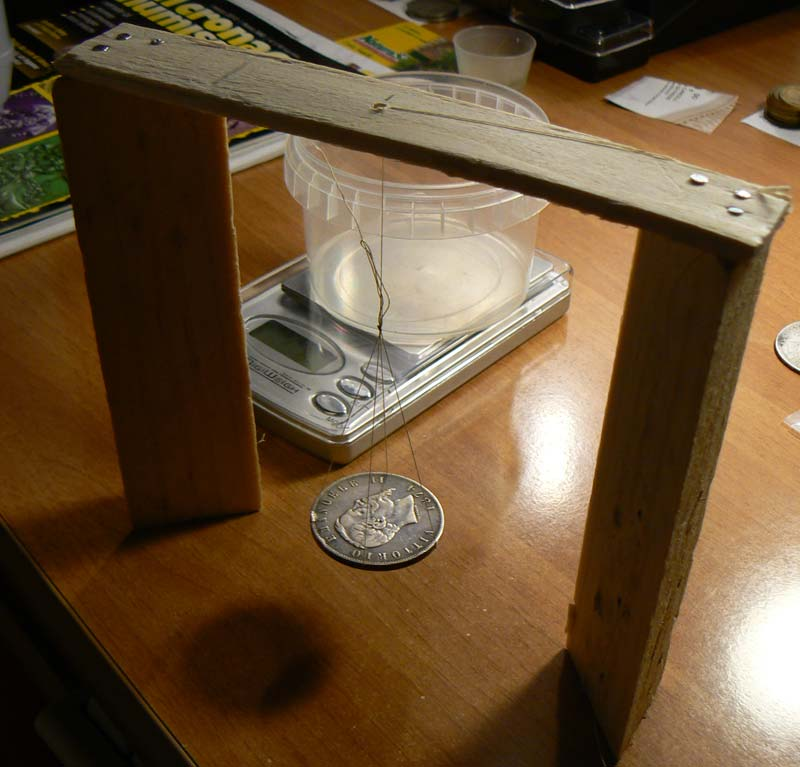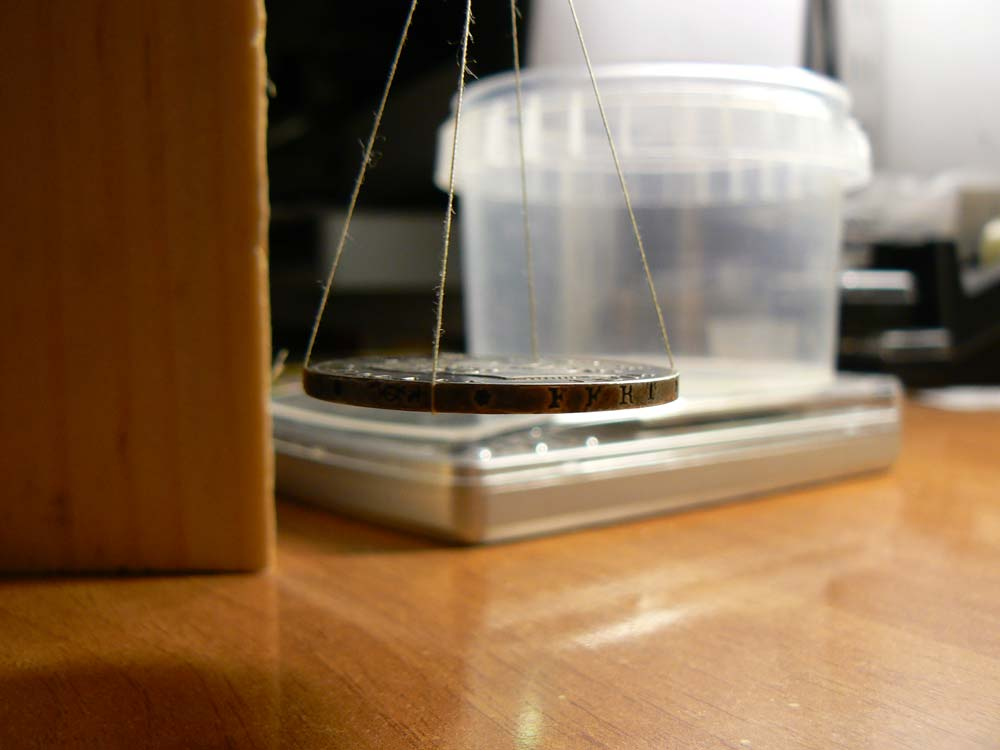At this point we can check the built suspension system and we can adjust the length of the wire.
We need to get a small can of clear plastic (must be light and transparent).
In these two pictures below we can see the coin into the jar suspended and in the second picture, especially, we can see the coin that does not touch any wall of the jar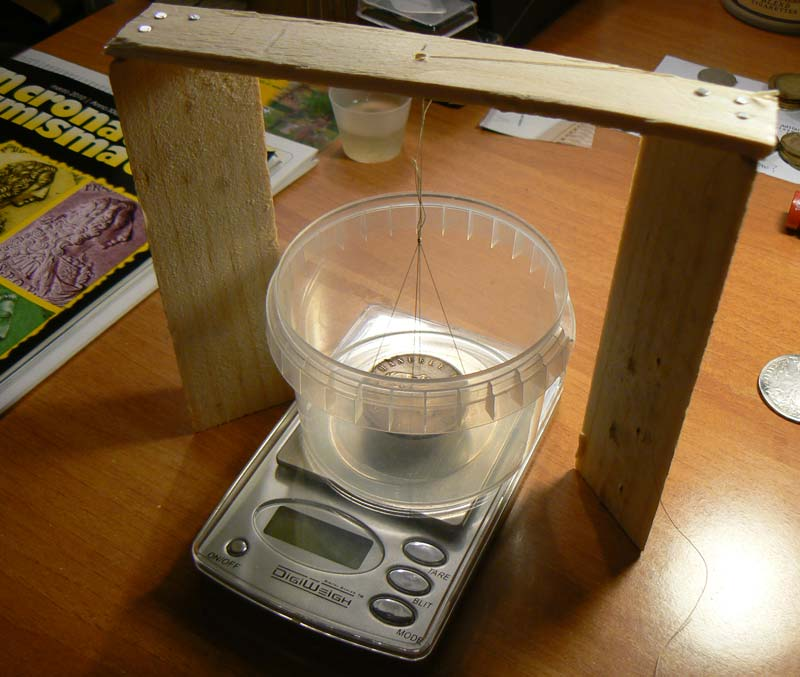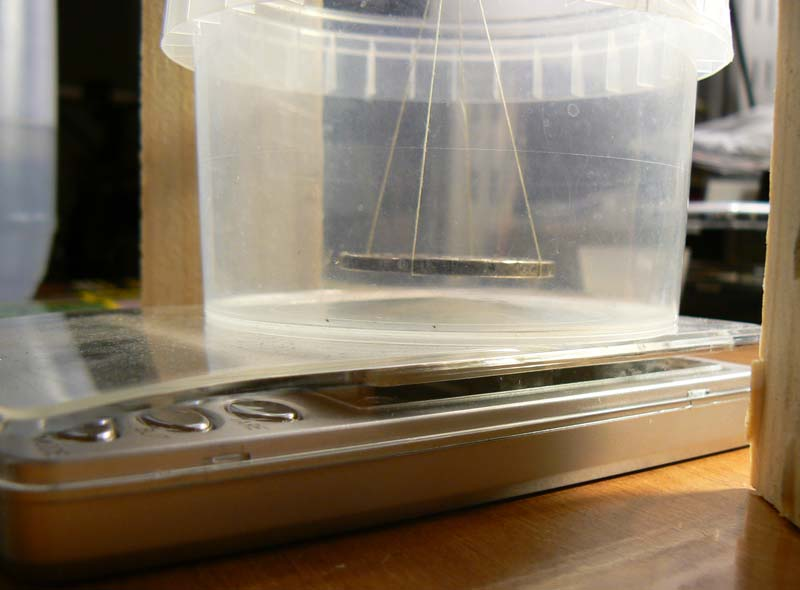At this point we can continue with the following steps:
• 5 - add distilled water in the jar (washed and cleaned if possible), it is important to use distilled water because it it a liquid which is known exactly the specific gravity. It is possible to use other liquids but we have also different calculations.
• 6 - weight the jar with water taking care not to go over the capacity of the digital scale.
• 7 - tare the scale to 0 (Figure below)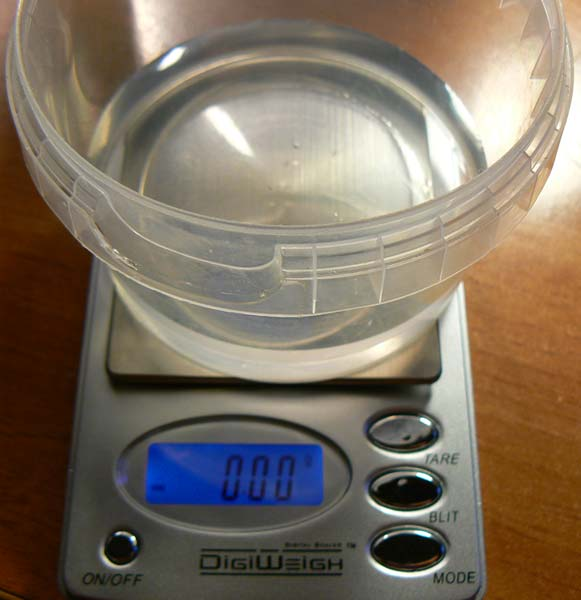• 8 - put the suspension system into the jar
at this stage we must take care that no air bubbles stay under the coin, it can be a bit laborious, maybe try to drown the coin a bit tilted, taking care not to touch the water with your fingers (the penalty it the failure of the experiment)
you can see in the figure below the coin immersed in water and the reading of the weigh.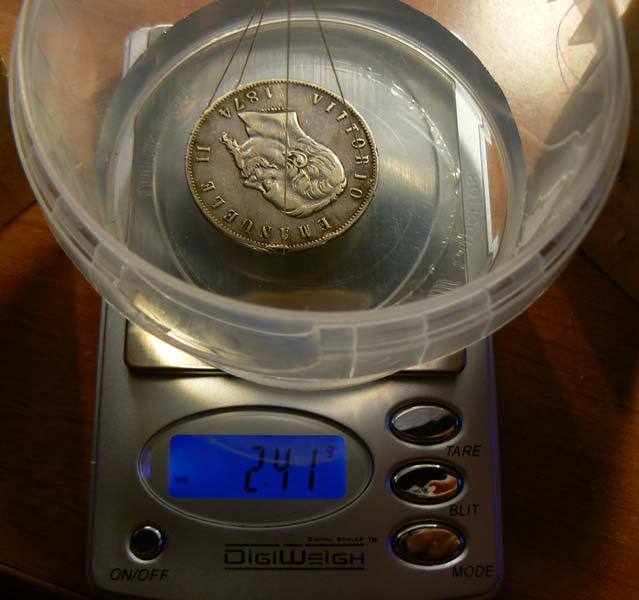06

The following image is my favourite, as you can see there are no bubbles under the coin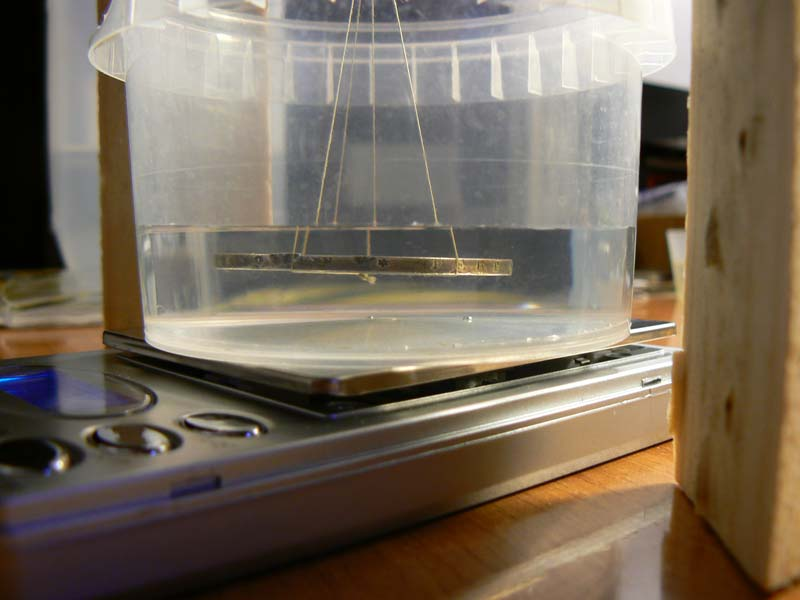Now we're almost there! a bit of math will be with us until the end.
The extra weight of water with the coin submerged is: 2.41 grams (the weight of the volume of water moved from the coin (and wire))
given the accuracy of the scale we can assume that the last weight is between 2.405 g and 2.415 g. The same the weight of the coin we can consider between 24.885 and 24.895
will be useful to calculate the error.

Note: We are calculating the specific gravity, since we use distilled water that has the same value of density (gt/cm3) if we use another liquid the calculations are different.

### Result

Well now we simply do
Coin dry weight / weight of water that have the volume of the coin = 24.89 / 2.41

specific gravity of our coin = 10.3278 g/cm³

Specific gravity of silver 900 (Latin Monetary Union):
Silver: 0.01049 g / mm ³
Copper: 0.00893 g / mm ³
Silver 900: 0.010310 g / mm ³ = 10.310 g/cm³

We have achieved something very precise:

percentage difference in measure: 0.17%
We can now estimate the maximum error in the calculation starting from the know error for the measure of weight:
Specific Gravity Minimum 10.3043 and maximum 10.3514
error ± 0.2%

### Considerations on accuracy

The calculation of a specific gravity of a big can be carried out, as we have seen, with a digital scale that have an accuracy of 0,01 grams.
Such kind of digital weight can be used with coins that weight more than 5 grams.

In table below it is possible to see the errors made using a digital scale with an accuracy of 0,01 grams when calculating silver coins with a purity of 900, so with a specific gravity of 10,33 (see next tables)
 Weight Coin SGmin SGmax ERR 24,89 10,304 10,351 0,23% 12,45 10,281 10,375 0,45% 6,22 10,235 10,423 0,90% 3,11 10,143 10,519 1,79% 2,51 10,099 10,566 2,21% 1,25 9,880 10,814 4,34% 0,63 9,466 11,344 8,34%
As it is possible to see an error of 1% can give a different specific gravity, up to 10% !
In table if a coin with a weight of 6,22 grams is taken than it is possible to find purity from 835 to 980: too much.
For example below the result of some measurement carried out on some Italian coins of 5 lire of Vittorio Emanule III that have purity of 835 and a weight of 5 grams. In chart below the squared dots are the result of experiment carried with a 0,01 digital scale.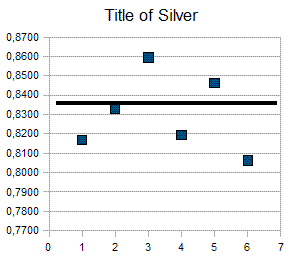So if we want evaluate the specific gravity of a Roman denari or a gold zecchino we have to use a digital scale with an accuracy of 0,001 grams.
In table below are reported the errors we can have with a 0,001 digital scale and with silver coin that have a purity of 900/1000 and so should have a specific gravity of 10,33 (see next tables)

 Weight Coin Pmin Pmax ERR 24,89 10,3255 10,3302 0,02% 12,45 10,3231 10,3325 0,05% 6,22 10,3184 10,3372 0,09% 3,11 10,3090 10,3466 0,18% 2,51 10,3045 10,3512 0,23% 1,25 10,2814 10,3746 0,45% 0,63 10,2353 10,4218 0,90%

As we can see we can have great accuracy also for "small" coins.

### General SG of some alloy

SG of some metals [Kg/dm³]
 Platinum 21.37 Silver 10.492 (10.40-10.53) Gold 19.32 Copper 8.93 lead 11.24 Zinc 7.13 (7.04-7.16) Tin 7.29 Nikel 8.02 Cadmium 8,648

For Alloy we have to calculate the percentage in weight of the mail metal against the other alloy composition.
For example if a SG of a denarius of Titus is found to be 10.4, meaning it contains 94% silver and 6% copper and impurities.

SG of some alloys, calculated values (Gold with copper, Silver with copper, Copper with Zinc and Copper with Tin)
 Purity 100% Purity 98% Purity 90% Purity 835% Purity 75% Purity 50% Gold 19.30 18.86 17.29 16.20 14.96 12.21 Silver 10.49 10.45 10.31 10.20 10.05 9.65 Copper (+Zinc) 8.93 8.89 8.71 8.57 8.40 7.93 Copper (+Tin) 8.93 8.89 Bronze 8.73 Bronze -asses 8.61 Orichalcum - Sestertii and dupondii 8.46 White alloy used for many fakes 8.03

Other information can be found in
'The Specific Gravity of the Gold Coins of Aksum' by W. A. Oddy and S. C. Monro-Hay in 'Metallurgy in Numismatics' by Metcalf and Oddy, 1980.
This paper showed
gold of 94% = SG of 18.5,
92% = SG 18.15,
91% = SG of 18.01.
Also 'The Chemical composition of Parthian Coins' by E. R. Caley, chapter VII in 'Numismatic Notes and Monographs' #129, ANS, 1955, which has a Table XXIV, of silver content related to SG. Some figures from that chart are;
99% silver = SG 10.48,
95% = 10.41,
86% = 10.24,
75% = SG 10.05.
Thanks to Marvin of Moneta-L for this data.

Plese remember that in many gold coins the know gold content in the alloy can be found to be with an error of +/- 2,0%.

### Possible errors in the experiment

weight of the wire:
we demonstrated that the weight of the wire give an error less than 0.0005 grams

specific gravity of water:
the specific gravity of a body changes with temperature, the specific weight of distilled water at 4 degrees is 1.0000 g/cm³
the specific gravity of water at 20 degrees is 0.9982 g/cm³
there is therefore an error of about ± 0.18% in the calculation, although we can correct it only by calculation

for maximum accuracy, the coin should be cleaned and degreased, as is feasible without major problems on gold is not on silver coins that could be ruined if patina is taken away.

becomes difficult to resolve the problem mathematically in the case of three or more metal alloys, the only viable solution is the use of XRF spectrometry (X-ray Fluorescence) (but that give indication of alloy composition only of the most surface layer of the coin). With an alloy of three metals, if we do not know any of the three ratios we should solve two equations with three unknowns and this is not possible, however, we could take same assumptions and calculate the possible error to see how this could affect the result.

For many ancient silver coins like antoninianus, Alexander tetradracms, etc. namely coins that are not in simple binary alloy, the result would be just a number to be interpreted, and can be used at least, for comparative tests.
Also for Republican and Imperial denarii sometimes you might make a wrong measure. While ignoring the trace of other elements, should be considered, as example, that a lot of denarii may contain gold percentages to 0.7-0.9% and in other cases lead to over 6%. For example
denariA: Ag 90% - Cu 10% = specific gravity 10.310 g/cm³ (theoretical)
denariB: Ag 80% - Cu 13% - Pb 7% = specific gravity 10.304 g/cm³ (theoretical)
In practice, the results must always be interpreted and not used as the verdicts on the coins analysed.

If you analyse gold and silver having a high title, say above 95% then the results can be accurate and can give us a good indication of the alloy of the coin. Gradually, the title of principal metal decreases the results are increasingly inaccurate. Coins that have low gold content would not give such great signs from their specific gravity because we do not know the proportion of other metals in the alloy.

### Conclusion:

The proposed method it is useful to calculate with good accuracy the specific gravity of a coin, applies to antique and modern coins, and it is not at all destructive.
A really useful tool in the hands of enthusiasts and professionals to get a good indication of the specific gravity of the coin that is going to be studied!

Alessandro Attila the collector

### Note

#### Use of another liquid for the measure.

to avoid generation of bubbles in our weight experiment we can use a different liquid insted demineralized water. We have to keep in account the different specific gravity of the new liquid we are going to use.
with the following notations
Pm weight of the coin
Vm volume of the coin = volume of liquid moved by the coin body
Pq weight of the liquid with the same volume of the coin
Psq Specific gravity of the liquid
in a case of a generic liquid we have

Psm = Pm /Vm

weighting the liquid moved by the body of the coin:

Vm = Pq / Psq

so

#### Psm = Pm * Psq / Pq

so we can see why when we use distilled water with a specific gravity equal to 1 the formula became Ps = Pm / Pq
if for example we use ethanol at 95% that have a specific gravity of 0,81 the formula to use get the following:
Ps = Pm * 0,81 / Pq

A different solution is to wet the coin in alcohol, the adherence of alcohol to coin is, we would dare to say, far less than volume of the thread that hangs the coin. On the other side, alcohol mixes with water, and is only measured the volume (well, weight) of coin.

One could use indeed ethanol, but that involves some troubles, like it is very sensitive to increases/decreases in temperature

Also we could use de-mineralized water with a drop of tensioactive, being the best dioctylsodiumsulfosuccinate (aerosol OT is a commercial brand). But that is not that easy to get.

Finally we can simply add a drop of the dishwasher we use generally.

#### Use of a little dish for the weight:

we could use a little transparent dish where we could place the coin we are going to weight. We always be careful to avoid bubbles between dish and coin, for little coins can be useful to submerge before the little dish and then "diving" over the coin being careful do not touch the water.

### Note on specific gravity of a alloy

we assume that the specific gravity of alloy composition is given on mass and not in volume. This is not correct but in case of two metals the error is negligible.
If we assume that a coin is made of two metals we could calculate the title.
For example:
is X the silver tile of a coin made with silver and copper
is P and V the coin weight and volume
is Ps the specific gravity, measured with our system, of the coin
Parg and Pram the weights respectively of silver and copper in the coin.
Psarg and Psram the specific gravity respectively of silver and copper.
then

P = Parg + Pram = Psarg * Varg + Psram * Vram = Parg * V * X + Psram * V * (1-X)
so considering P/V =Ps
Psarg * X + Psram * (1-X) = Ps
that with simple maths operations

X = (Ps-Psram)/(Psarg - Psram)

### Note attached: demonstration that from calculation of diameter and thickness we do not get the volume of the coin

I take one of the Scudo coin I have and I measure the following data:
Diameter: 37 mm
Thickness: 2.5 mm

The thickness was measured at the edge with a micrometer that appreciates the tenth of a millimetre.
Unfortunately I can not measure well the thickness at the fields but I note that the thickness at the field is near to 2.35 mm

Now:
the surface of the coin is 3.14 · D² / 4 = 1075.21 mm²
then

Volume = 2688.03 mm ³

Well at this point I report the specific gravities of the content metals of the coin:
Silver: 0.01049 g / mm ³
Copper: 0.00893 g / mm ³

Please note however that the specific gravity of alloy composition is given on mass and not in volume. Ie every 100 grams of Ag900 90 grams are silver and 10 grams copper. If we make the calculation of the specific gravity with volume ratio we would have an approximation because the assumption that the total volume of the alloy is equal to the volume of the two separated metals is not always true, however in this case the error would be not so high. In any case if we do the calculation using volume ratios we reach a very close result, it is not the sore point.
With a bit of calculations by solving two equations with two unknowns (omitted here)

our result:
Volume occupied by silver: 2377.69 mm ³
Volume occupied by copper: 310.34 mm ³

Multiply the Specific Gravity for the volume just found:
SG x Volume occupied by silver = weight of silver
Silver weight: 24.94 g
Copper weight: 2.77 g

The sum is 27.71 grams

Ok ok, you can say that the problem is simply the measure of the thickness of the coin. Probably, but I did the calculation for the correct value and we should measured a thickness of 2.255 mm but it would be too much, even below the surface of the field, to try again I did this analysis with 5 Lira 1927 little eagle Italy and the result is that I should have a thickness of 1.18 mm instead of 1.68 correctly measured (we are really out here)

then this technique is wrong and can not be used for the calculation of a coin volume.

Alessandro Attila the collector

Bibliograpy

Home of Attila Coin Site

Users online now: 63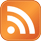Most Recent Articles.Most Popular ArticlesAuctionsEventOff topicPrecious MetalsSite UpdateStores
..